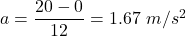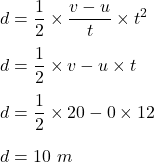## A car takes 12 s to go from v=0m/s to v = 20 m/s at constant acceleration. Part A If you wish to find the distance traveled using the equati

Question

A car takes 12 s to go from v=0m/s to v = 20 m/s at constant acceleration. Part A If you wish to find the distance traveled using the equation d=1/2at2, what value should you use for a?

in progress 0
2 months 2021-07-30T19:49:12+00:00 1 Answers 6 views 0

a = 1.67 m/s²

Explanation:

Given that,

Initial speed, u = 0

Final speed, v = 20 m/s

Time, t = 12 s

We need to find the distance traveled using the equation, d = 1/2 at².

Where

a is acceleration, a = (v-u)/t,So,Hence, the acceleration is 1.67 m/s² and the distance traveled is 10m.